# Chemical reactions

Chapter overview

1 week

This chapter builds on the introduction to chemical equations given in Chapter 1 and 3 of Gr. 8 Matter and Materials.

By this stage, learners should know that atoms are rearranged during a chemical reaction. The atoms do not change; only their arrangement in relation to each other changes.

Learners were introduced to particle diagrams in Gr. 8 and this skill will be further reinforced in this chapter. We have tried to introduce learners to the idea that chemical reactions can be thought of in different ways. Ultimately, they have to be able to write chemical equations, but this is a very complex skill. By starting with word equations and progressing to submicroscopic representations (picture equations) before translating the latter to the symbolic format (chemical equations), we hope to build/scaffold the learning of chemical equations as well as develop learners' ability to imagine events on the submicroscopic scale.

2.1 Thinking about chemical equations (0.5 hours)

 Tasks Skills Recommendation Activity: Drawing water Drawing Optional (Suggested)

2.2 How do we represent chemical equations? (1 hour)

 Tasks Skills Recommendation Activity: Identifying the different types of equations Identifying, sorting and classifying, interpreting Optional (Suggested)

2.3 Balanced equations (1.5 hours)

 Tasks Skills Recommendation Activity: When is a reaction balanced? Interpreting, writing formulae, balancing equations Optional (Suggested) Activity: Magnesium burning in oxygen Interpreting, writing formulae, balancing equations CAPS suggested Activity: Iron reacts with oxygen Interpreting, writing formulae, balancing equations, comparing CAPS suggested Activity:Copper reacts with oxygen Interpreting, writing formulae, balancing equations, comparing, drawing CAPS suggested

• What is a chemical reaction?
• How can we represent what happens in a chemical reaction?
• What do the different symbols in a chemical reaction equation mean?
• What do the numbers in a chemical reaction mean?
• What does it mean to balance a chemical equation?
• How can we tell if a reaction is balanced?
• How do we translate between word equations, picture equations and chemical equations?

In Gr. 8 Matter and Materials we learnt about chemical reactions for the first time. Can you remember the main ideas about chemical reactions? Here they are again:

• During chemical reactions, materials are changed into new materials with new chemical and physical properties.
• The materials we start with are called reactants, and the new materials that form are called products.

• During a chemical reaction, atoms are rearranged. This requires that bonds are broken in the reactants and new bonds are formed in the products.

In this chapter we are going to build on these ideas. We will focus on two things:

1. how to write chemical reaction equations; and
2. how to balance chemical reaction equations.

This will prepare us for the chapters that follow this one, in which we will be looking at different types of chemical reactions.

Before we get to chemical reactions, however, it is important that we remind ourselves of the different ways that we have been thinking about chemical compounds up to now. The next section will show how they all fit together.

• bond
• reactant
• product
• chemical reaction
• macroscopic
• submicroscopic
• symbolic

Scientists learn to think about compounds on three different levels:

• macroscopic
• microscopic
• submicroscopic

As a young scientist, you have already been introduced to this kind of thinking. The three levels can also be thought of as three different ways to represent compounds. The next activity will help you understand what this means.

# Drawing water

INSTRUCTIONS:

The instruction for this activity is really simple: Draw a picture of water. You may use the space below for your drawing.

Do not give any further instructions, but allow the learners to interpret the question in any way they want to. Ask the learners to show their work. Some may draw a landscape with water (a dam or river) and others may draw a glass or similar container with clear colourless liquid inside it. Perhaps one or two will draw a water molecule or the chemical formula for water. Get some of the learners to redraw their pictures on the board.

Your drawing may look like one of the diagrams below. They all represent water. But which one is correct?

They are all correct!

The three diagrams above all represent water, but they are very different from each other. We say that they are three different representations of the same thing, namely water.

The following diagram shows how the three representations fit together.

The water molecule in the top right shows what a particle of water would look like (i). We cannot see water particles with our eyes, therefore we have to imagine them. This is why the water molecule is inside a thought bubble. We call this a submicroscopic representation.

Submicroscopic means 'smaller than microscopic', or 'too small to see through a microscope'. Sometimes it helps to think that this is what we would see if we had special 'submicroscopic goggles' on to 'see' at the atomic level!

Find out about special microscopes which enable people to 'see' atoms http://myscienceacademy.org/2013/04/03/have-you-ever-seen-an-atom/

The beaker of water shows what water looks like to our eyes (ii). We call this a macroscopic representation, because it is observable. That means it can be observed by using our senses such as seeing, feeling, hearing, tasting or touching.

The chemical formula on the left uses chemical symbols to represent water (iii). We have learnt that chemical formulae are made up of element symbols. We can think of chemical symbols and formulae as a chemical 'language', because they tell a story. The 'story' told by the formula H2O is that a water molecule consists of two atoms of H and one atom of O. The formula 'H2O' is a symbolic representation.

Experienced scientists can move easily between these three levels. They can translate the symbolic language of chemical formulae to submicroscopic pictures in their mind. This is what we will practice in this chapter.

Before moving on, try another example where you draw the 3 different levels of carbon dioxide in the space below. label each level.

Learners could draw a closed container with a clear gas in it for the macroscopic representation. They should write the formula CO2 for the symbolic representation.They should draw a carbon dioxide molecule for the submicroscopic representation as follows:

The world's smallest movie made with atoms and how the scientists made it

## How do we represent chemical reactions?

• chemical equation
• coefficient
• subscript

 reactants, products, bonds, rearranged, atoms, molecules, new compounds

Get learners to first take some notes and describe what they think a chemical reaction is. You can even just ask them the question and get their definitions.

A chemical reaction is a rearrangement of atoms in which one or more compounds are changed into new compounds.

All chemical reactions can be represented by equations and models. To some people, chemical equations may seem very hard to understand. Since atoms and molecules can not be seen they have to be imagined and that can be quite difficult! Luckily, we have had some preparation because we have been drawing molecules since Gr. 7.

Anytime that atoms separate from each other and recombine into different combinations of atoms, we say a chemical reaction has occurred. No atoms are lost or gained, they are simply rearranged.

### 1. Word equations

In mathematic equations we use an equal sign (=) for example 2 + 2 = 4, but in scientific chemical equations, we use an arrow (→), for example C + O2→ CO2.

When we represent a chemical reaction in terms of words, we write a word equation. For example, when hydrogen gas reacts with oxygen gas to form water, we can write a word equation for the reaction as follows:

hydrogen + oxygen→ water

To the left of the arrow, we have the 'before' situation. This side represents the substances we have beforethe reaction takes place. They are called the reactants. What are the reactants of this reaction?

The reactants are hydrogen and oxygen.

To the right of the arrow we have the 'after' situation. This sides represents the substances that we have after the reaction has taken place. They are called the products. What is the product of this reaction?

The product is water.

### 2. Picture equations

The same reaction of hydrogen reacting with oxygen, can also be represented in pictures called submicroscopic diagrams. The diagram below shows that the atoms in two hydrogen molecules (H2) and one oxygen molecule (O2) on the left rearrange to form the two water molecules (H2O) on the right of the arrow. Hydrogen atoms are white circles and oxygen atoms are red circles.

What kind of representation is this: macroscopic, submicroscopic, or symbolic?

Submicroscopic, because it shows the particles.

Now we are going to convert our submicroscopic picture to a symbolicone:

What is the product of the above reaction? What are the reactants of the above reaction? Write their formulae.

The product is H2O. The reactants are H2 and O2.

### 3. Chemical equations

When we represent a chemical reaction in terms of chemical formulae (symbols), it is called a chemical equation. The chemical equation for the above reaction would be as follows:

2 H2 + O2→2 H2O

What kind of representation is this: macroscopic, submicroscopic, or symbolic?

Symbolic, because it uses formulae (symbols).

We still have reactants on the left and products on the right.

# Identifying the different types of equations

INSTRUCTIONS:

Complete the following table by identifying the different types of equations which have been shown, namely word, picture or chemical equations.

 Equation Type of equation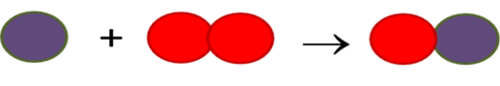carbon dioxide + water → glucose + oxygen Fe + O2→ Fe2O3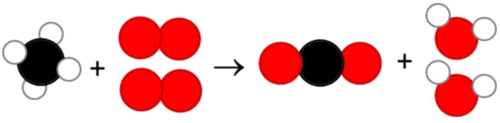C6H12O6 + 6O2→ 6CO2 + 6H2O

 Equation Type of equation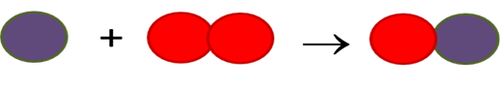Picture equation carbon dioxide + water → glucose + oxygen Word equation Fe + O2→ Fe2O3 Symbolic/chemical equation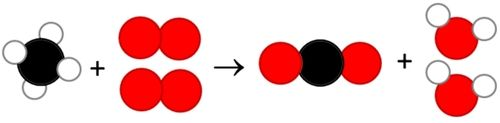Picture equation C6H12O6 + 6O2 → 6CO2 + 6H2O Symbolic/chemical equation

QUESTIONS:

What process does the equation, carbon dioxide + water → glucose + oxygen, represent?

Photosynthesis.

What process does the equation, C6H12O6 + 6O2 → 6CO2 + 6H2O, represent?

Cellular respiration.

When you look at the reaction equation above you will notice two kinds of numbers:

• Numbers in front of chemical formulae in the equation. They are called coefficients.

• Smaller numbers used inside and below the chemical formulae. These are called subscripts.

Coefficients and subscripts mean different things, as you will see in the next section.

### Coefficients and subscripts in chemical equations

Why is there a '2' in front of the formula for water (H2O) in the chemical equation for water? This is because two molecules of H2O can be made from two molecules of H2 and one molecule of O2 in our reaction.

The numbers in front of the formulae in the chemical equation are called coefficients. They represent the numbers of individual molecules that are in the chemical reaction.

You will notice that O2 does not have a coefficient in the reaction above. When there is no coefficient, it means that just one molecule of that substance takes part in the reaction.

In the previous chapter, we learnt how to interpret chemical formulae. When we read the formula, the subscripts tell us how many atoms of a particular element are in one molecule of that compound.

## Balanced equations

• balanced
• chemical formula
• rust
• tarnish

Now we are going to learn what it means when a reaction is balanced. Here is our submicroscopic picture again.

When learners draw a diatomic molecule, the two atoms must be touching to show that they are chemically bonded, otherwise it is wrong.

Count how many H atoms are on the left side of the reaction. How many on the right?

Four H atoms on the left and four H atoms on the right.

Count how many O atoms are on the left side of the reaction. How many on the right?

Two O atoms on the left and two O atoms on the right.

Did you notice that the numbers and types of atoms are the same on the left and on the right of the reaction? The reactants have four H atoms and two O atoms. The products have four H atoms and two O atoms.

When this is true of a reaction equation, we say the equation is balanced.

# When is a reaction balanced?

INSTRUCTIONS:

1. Study the equation below. The black atoms are carbon (C), and the red atoms are oxygen (O). They will not always necessarily be this colour - this is just a representation.
2. Answer the questions that follow.

QUESTIONS:

What kind of representation is this: macroscopic, submicroscopic or symbolic?

Submicroscopic, because it shows the particles.

Write a symbolic representation (a chemical equation) for the above reaction.

C + O2→ CO2

Write the formulae for the reactants of this reaction.

C and O2

Write the formula for the product of the reaction.

CO2

Count how many C atoms are on the left side of the reaction. How many on the right?

One C atom on the left and one C atom on the right.

Count how many O atoms are on the left side of the reaction. How many on the right?

Two O atoms on the left and two O atoms on the right.

Is the reaction balanced? Why do you say so?

Yes, the reaction is balanced because equal numbers of the same kinds of atoms are on both sides of the reaction equation.

Now that we know how to recognise a balanced equation, we are going to learn how to balance them!

What is a balanced equation? Write down your own definition.

Either get learners to write down their own definitions and then read them out to the class, or they can just volunteer answers. You can get learners to start with: We say an equation is balanced when... A potential answer is: "We say a reaction is balanced when the total numbers and types of atoms in the reactants are equal to those in the products".

We are going to use a few examples of real reactions to learn how to balance equations. In the chapters following this one, we are going to see what these reactions look like in real life, but for now, we will just focus on how to balance equations.

# Magnesium burning in oxygen

Magnesium flakes are often used in some fireworks, such as sparklers, because when they burn they create the bright, shimmering sparks.

When magnesium metal burns in oxygen, we can write the following word equation for the reaction that occurs between these two elements:

magnesium + oxygen→ magnesium oxide

QUESTIONS:

What are the reactants of the reaction?

The reactants are magnesium and oxygen.

What is the product?

The product is magnesium oxide.

We can change the word equation into a chemical equation:

Mg + O2 →MgO

What kind of representation is this: macroscopic, submicroscopic, or symbolic?

Symbolic, because it uses formulae (symbols).

Is the equation balanced? If you are not sure, count the number of each type of atom on the left, and on the right. Perhaps it will help to look at a submicroscopic representation (a particle diagram) of the reaction:

You can write your results in the table below:

 Number of atoms Reactants Products Mg O

 Number of atoms Reactants Products Mg 1 1 O 2 1

No, the equation is not balanced because the numbers of the atoms are not the same in the reactants and products.

So how could we balance the equation to describe magnesium burning in oxygen? When balancing reactions, there is one simple rule:

You may only add compounds that are already in the equation. This means only coefficients may be changed, not subscripts!

Let's try a few alternative solutions. Would it help to add an O atom on the right, like this?

Now the O atoms are balanced on both sides of the equation, but we don't have MgO on the right anymore. We have changed the formula on the right to MgO2. That means we have changed a subscript in the formula. You cannot change the formula of a compound when balancing chemical equations.

Ask learners why it is not allowed. Is MgO the same as MgO2? Remind them of the earlier example of H2O and H2O2, which were not the same compound. MgO and MgO2 can not be the same compound because they do not have the same chemical formula. The ratio of Mg and O atoms are different in the two compounds. (The compound MgO2 does not even exist, but you do not have to go into this.)

Adding single atoms to any side of the equation is also not allowed. That means the following equation is also not correct:

Remember that we may only use the chemical formulae that are already in the equation. We need two MgO's on the right to balance the two O's in O2. We also need two Mg's on the left to balance the two MgO's on the right.

Can you build this equation with play dough balls or beads? When you convert the play dough 'reactants' to 'products', are there any unused 'atoms' left behind afterwards?

No, there are not. Get learners to actually do this activity and practice making the equation using balanced numbers of atoms.

Now, let us take this a step further. We are going to convert our balanced submicroscopic equation to a symbolic chemical equation. Write down a balanced equation for magnesium burning in oxygen to produce magnesium oxide.

Write this up on the board and explain again how the equation is balanced:

2 Mg + O2→ 2 MgO

Here are a few important rules for balancing chemical equations:

• When we balance reaction equations we may ONLY add coefficients to the chemical formulae that are already in the equation.
• We may NOT change the chemical formulae of any of the reactants or products by changing the subscripts in a formula.
• We may NOT add other reactants or products. This includes adding single atoms of any of the elements already in the reaction equation.
• We may NOT remove reactants or products.

We are now ready to practice balancing other reaction equations.

# Iron reacts with oxygen

When iron rusts, it is because the iron metal reacts with oxygen in the air to form iron oxide.

The word equation is the following:

iron + oxygen→ iron oxide

The chemical equation is the following:

Fe + O2→Fe2O3

Is the equation balanced? Draw a submicroscopic picture to help you decide.

Learner's diagram should look like this. They may find it difficult to convert the equations into diagrams. Help them to interpret the formulae in the following way: Fe on its own means there is just one atom of iron (Fe). O2 means there must be two atoms of O, linked up to form a molecule. Fe2O3 means two Fe atoms and three O atoms are clustered together.

The colours are not important, as long as all the atoms of the same element are the same colour. The arrangement of the atoms in the Fe2O3 'cluster' is also not important. Since Fe2O3 is an ionic compound, we would not ordinarily speak of a 'molecule' of Fe2O3. Like all other ionic compounds, it consists of large clusters of Fe3+ and O2- ions in a regular crystalline packing that extends in three dimensions, much like the ionic lattice of NaCl in the picture below (shown in Chapter 1 also).

It is not recommended that you mention this information here, as it more likely to confuse learners at this point than add to their understanding of balancing equations.

You could also use a table like the one below:

 Number of atoms Reactants Products Fe O

 Number of atoms Reactants Products Fe 1 2 O 2 3

No, the equation is not balanced because the numbers of the atoms are not the same in the reactants and products.

How could we balance the reaction? Three possibilities (Plans A, B and C) are given below. You must evaluate each plan, and say if it is allowed or not.

Plan A

 Changes made Is this change allowed? Yes/no? Reason Add one Fe atom on the reactant side. Change O2 to O3 on the reactant side of the equation.

 Changes made Is this change allowed? Yes/no? Reason Add one Fe atom on the reactant side. Yes The Fe atom is already a reactant. Change O2 to O3 on the reactant side of the equation. No Changes to formulae are not allowed.

Convert the picture equation above to a chemical equation.

2 Fe + O3→ Fe2O3

Did any coefficients change? Remember that this is allowed.

Yes.

Did any formulae change, or were any new formulae added? Remember that this is NOT allowed.

Yes.

No, because a formula was changed.

Plan B

 Changes made Is this change allowed? Yes/no? Reason Add one Fe atom on the reactant side. Add one O atom on the reactant side.

 Changes made Is this change allowed? Yes/no? Reason Add one Fe atom on the reactant side. Yes The Fe atom is already a reactant. Add one O atom on the reactant side. No Adding single atoms is not allowed, unless they are already in the reaction AS SINGLE ATOMS.

Convert the picture equation to a chemical equation.

Two Fe + O + O2→ Fe2O3

Did any coefficients change? Remember that this is allowed.

Yes.

Did any formulae change, or were any new formulae added? Remember that this is NOT allowed.

Yes.

What do you think: Can this plan work? Explain why or why not.

No, because adding formulae is not allowed.

Plan C

 Changes made Is this change allowed? Yes/no? Reason Add three Fe atoms on the reactant side. Add two O2 molecule on the reactant side. Add one Fe2O3 on the product side.

 Changes made Is this change allowed? Yes/no? Reason Add three Fe atoms on the reactant side. Yes The Fe atom is already a reactant. Add two O2 molecule on the reactant side. Yes The O2 molecule is already a reactant. Add one Fe2O3 on the product side. Yes Fe2O3 is already a product.

Convert the picture equation to a chemical equation.

4 Fe + 3 O2→ 2 Fe2O3

Did any coefficients change? Remember that this is allowed.

Yes.

Did any formulae change, or were any new formulae added? Remember that this is NOT allowed.

No.

What do you think: Can this plan work? Explain why or why not.

Yes, because none of the rules for balancing equations were broken.

Which of the three plans (A, B or C) helped us to balance the equation using only moves that are allowed?

Plan C

Are there any other plans that you can think of to balance this equation?

Learners should be encouraged to try out other possibilities. They should come to the conclusion that the reaction proposed in Plan C is the only correct one.

In the next activity we will balance an equation that is much simpler, but we are not going to include all the explanations of the previous activity.

# Copper reacts with oxygen

Have you ever noticed how copper items tarnish over time?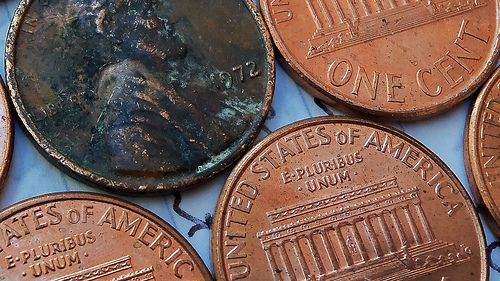One of these copper coins is tarnished as it has become coated in a black substance. http://www.flickr.com/photos/dno1967b/6996590307/

This dark layer of tarnish is the result of a slow reaction between copper and oxygen, to form copper oxide.

QUESTIONS:

Write the word equation for this reaction. The words are all in the sentence above, they just need to be placed in the correct positions.

Learners should fill in the answers as follows:

Convert the word equation into a chemical equation. You do not have to balance it yet.

Convert the chemical equation to a picture equation. It does not have to be balanced.

Learner's drawing should look similar to the following. Colours do not have to be the same as shown here, but they must show a difference between the different elements. If learners do not have different coloured pencils, they can also use different patterns to distinguish the different atoms.

Now, redraw the picture equation so that it is balanced. Remember that no 'new' compounds may be added; we are only allowed to draw more of the molecules that are already there.

convert the balanced picture equation to a balanced chemical equation.

In the chapters that follow, there will be more opportunities to write and balance chemical equations.

## Summary

• There are a number of different ways to represent chemical equations:

• With models and pictures (in submicroscopic representations);
• with symbols and formulae (in chemical equations); and
• with words (in word equations).
• Numbers are used in two different ways in chemical equations:

• Coefficients in front of chemical formulae indicate the numbers of atoms or molecules of a specific type that take part in the reaction; and
• Subscripts inside chemical formulae indicate the number of atoms of a specific type in that particular compound.
• Chemical reactions happen when atoms in compounds rearrange; no atoms are lost or gained during a chemical reaction.
• In a balanced equation equal numbers of the same kinds of atoms are on opposite sides of the reaction equation.

Concept map

The following concept map is incomplete. You need to describe when you get reactants and when you get products in a chemical reaction.

This is the completed concept map.

# Review questions

Why can we not change the subscripts in the formulas of reactants and products when we want to balance an equation? [2 marks]

This is because it will change the formula of the compound which then represents a different compound, and not the one involved in the reaction.

Write the balanced chemical equation between carbon and oxygen to form carbon dioxide. [1 mark]

C + O2→CO2

Write the balanced chemical equation between hydrogen and oxygen to form water. [1 mark]

2H2 + O2 → 2H2O.

Here is a balanced chemical equation: C + H2O→ CO + H2

Answer the four questions below that relate to this equation: [8 marks]

1. Write the formulae of the reactants of this reaction.

2. Write the names of the reactants of this reaction.

3. Write the formulae of the products of this reaction.

4. Write the names of the products of this reaction.

1. C and H2O. NOTE: Learners do not need to know this equation, it is just to practice balancing equations.

2. carbon and water

3. CO and H2

4. carbon monoxide and hydrogen

The balanced equation below represents the reaction between nitrogen monoxide (NO) and bromine (Br2): 2 NO + Br2→ 2 NOBr

Complete the table by counting how much of each atom is on each side of the reaction equation. [6 marks]

 Number of atoms In the reactants In the product Nitrogen (N) Oxygen (O) Bromine (Br)

NOTE: Learners do not need to know this equation, it is just to practice balancing equations.

 Number of atoms In the reactants In the product Nitrogen (N) 2 2 Oxygen (O) 2 2 Bromine (Br) 2 2

Turn the following chemical equations into word equations: [2 x 3 = 6 marks]

1. 2 CO + O2→ 2 CO2

2. 2 Mg + O2→ 2 MgO

1. carbon monoxide + oxygen→ carbon dioxide

2. magnesium + oxygen→ magnesium oxide

Turn the following word equations into chemical equations: [2 x 3 = 6 marks]

1. sulfur + oxygen→ sulfur dioxide

2. carbon monoxide + water→ carbon dioxide + hydrogen

1. S + O2→ SO2

2. CO + H2O→ CO2 + H2

Turn the following picture equations into chemical equations.[2 x 3 = 6 marks]

• The red circles represent oxygen (O) atoms.
• The white circles represent hydrogen (H) atoms.
• The grey circles represent carbon (C) atoms.
• The yellow circles represent sulfur (S) atoms.

1. 2 H2O→ 2 H2 + O2

2. 2 CO + O2→ 2 CO2

Write the following chemical equations as word equations: [4 x 1 = 4 marks]

1. 4 Fe + 3 O2→ 2 Fe2O3

2. What does this product look like?

3. 2 Mg + O2→ 2 MgO

4. What does the product look like?

1. iron + oxygen → iron oxide

2. It is a brown rusty coating (rust).

3. magnesium + oxygen → magnesium oxide

4. It is a white powder.

1. Turn the following chemical equations into picture equations: [2 x 4 = 8 marks]

CH4 + 2 O2→ CO2 + 2 H2O

figure id="gd-idp18317664">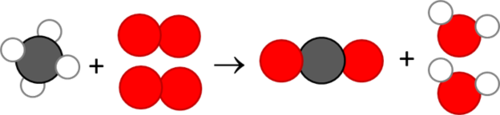CS2 + 3 O2→ CO2 + 2 SO2

Total [48 marks]